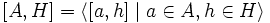Commutator of two subgroups is normal in join

Statement

Suppose$H, K$ are subgroups of a group$G$. Then, the Commutator of two subgroups (?)$[H,K]$ is a normal subgroup of the Join of subgroups (?)$\langle H, K \rangle$.

Facts used

1. Subgroup normalizes its commutator with any subset: If$H \le G$ is a subgroup and$A$ is a subset of$G$, then$H$ normalizes the following subgroup:$[A,H] = \langle [a,h] \mid a \in A, h \in H \rangle$

Here,$[a,h] = a^{-1}h^{-1}ah$ is the commutator of the two elements.

Proof

Given: Two subgroups$H, K \le G$.

To prove:$[H, K ] \triangleleft \langle H, K \rangle$.

Proof: By fact (1),$H$ normalizes$[K, H]$, which is the same as$[H,K]$. Also,$K$ normalizes$[H,K]$. Thus, the normalizer of$[H,K]$ in$G$ contains both$H$ and$K$, hence it contains$\langle H, K \rangle$, proving that$[H,K]$ is normal in$\langle H, K \rangle$.